Courses

# Signals And Systems - MCQ Test 2

## 20 Questions MCQ Test Mock Tests Electronics & Communication Engineering GATE 2020 | Signals And Systems - MCQ Test 2

Description
This mock test of Signals And Systems - MCQ Test 2 for Electronics and Communication Engineering (ECE) helps you for every Electronics and Communication Engineering (ECE) entrance exam. This contains 20 Multiple Choice Questions for Electronics and Communication Engineering (ECE) Signals And Systems - MCQ Test 2 (mcq) to study with solutions a complete question bank. The solved questions answers in this Signals And Systems - MCQ Test 2 quiz give you a good mix of easy questions and tough questions. Electronics and Communication Engineering (ECE) students definitely take this Signals And Systems - MCQ Test 2 exercise for a better result in the exam. You can find other Signals And Systems - MCQ Test 2 extra questions, long questions & short questions for Electronics and Communication Engineering (ECE) on EduRev as well by searching above.
QUESTION: 1

### If relation between input x[n] and output y[n] of a system is given by y[n] - 1/2 y[n -1] = x[n] then the system is

Solution:

Simply lake Z transform from both sides:

Then H(z)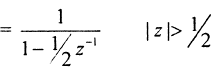it is right of the right - most pole

QUESTION: 2

### lf x[n] = - u[-n -1] + (1 /2)n u[-n -1] then ROC of signal is:

Solution: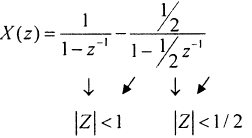Overall ROC is IZI < 1 /2

QUESTION: 3

### Consider, discrete time signal x[n] = (1 /3)n u[n] If signal y[n] is defined as y[n] = x[—n], —∞< n < ∞ then value of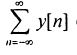equals to

Solution: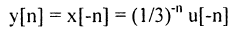Now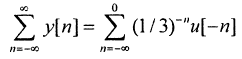Put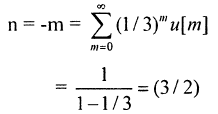QUESTION: 4

If signal x(t) and h(t) shown in figure are convolved to yield y(t).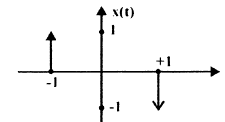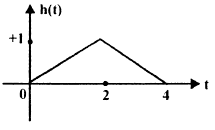Solution:

Simply use difference and sum between points.

QUESTION: 5

Given that g (t)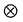g (t) = t exp (—t) u(t) then value of g(t) is

Solution: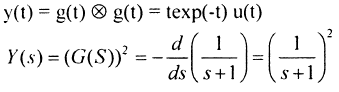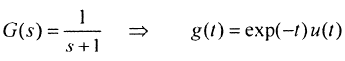QUESTION: 6

If u(t) r(t) denotes unit step and unit ramp respectively then u(t)r(t) denotes their convolution, then function u(t + 1)r(t — 2) is given by.

Solution:

y(t) = u(t)r(t)

Y(s) = 1/s3

QUESTION: 7

If wave form is given only for I period then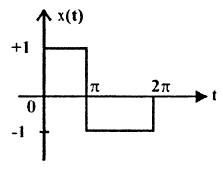Q. Waveform x (t) is

Solution:
QUESTION: 8

If wave form is given only for I period thenQ. Fourier series representation on of x (t) will be.

Solution:

it is an odd function with half symmetry.

QUESTION: 9

If complex Fourier- series coefficient Ck is given as Ck = (— 1/2)|k| then value of x(t) is:

Solution:
QUESTION: 10

Which statements are true about Fourier transform?

1. If x(t) is real then X(jω) is complex conjugate symmetric.
2. If x(t) is imaginary then X(jω) is complex conjugate anti-symmetric
3. If x(t) is even then X(jω) is also even.
Solution:
QUESTION: 11

Check status of linearity of given functions.

I .   x1(t) = x(t) .cost

2.  x2(t) = cos(x(t)).t

Solution:

x2(t) = t cos (x (t)

if x(t) = 0   ⇒  x2(t) = t

So x2(t) is non - linear

QUESTION: 12

If Fourier transform of x(t) is X(ω) then Fourier transform of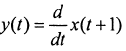Solution: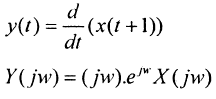QUESTION: 13

0.5 If Z-Transform X(z) of a sequence x[n] is given by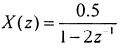.It is given that R.O.C. of X (z) includes unit circle then

Q. Value of x is

Solution: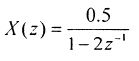QUESTION: 14

0.5 If Z-Transform X(z) of a sequence x[n] is given by.It is given that R.O.C. of X (z)includes unit circle then

Q. In above system if x[n] is causal then value of x is

Solution:

if it includes, unit - circle then, Iz| < 2

x[n] = - (0.5) 2n u(-n-1)

x = 0

if it is causal then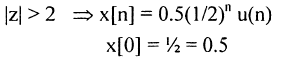QUESTION: 15

If frequency response of a causal & stable linear Time Invariant system is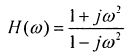, then value of phase delay and group delay are.

Solution: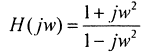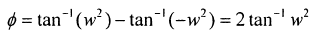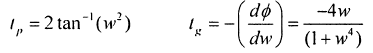QUESTION: 16

Which among the following are the stable discrete time systems?

1. y(n) = x(4n)
2. y(n) = x(-n)
3. y(n) = ax (n) + 8
4. y(n) = cos x(n)

Solution:
*Answer can only contain numeric values
QUESTION: 17

For given Fourier — series coefficients for real signals Co = 1, C1 = — 2, C2 = — 1 then power of this signal is _______

Solution:
QUESTION: 18

What is inverse Fourier transform of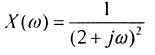Solution: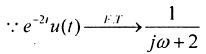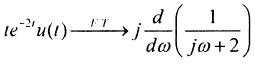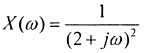QUESTION: 19

Which statement is true about y[n] = x[n] . cos (ωo n)

Solution:
*Answer can only contain numeric values
QUESTION: 20

For given signal s(t), shown below what is slope of matched filter output during interval 1 ≤ t ≤ 2 is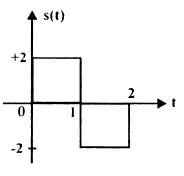Solution:

h(t) =    = s(2

y(t) = NWh(t )

Just draw y(t) and then calculate slope of y(t)